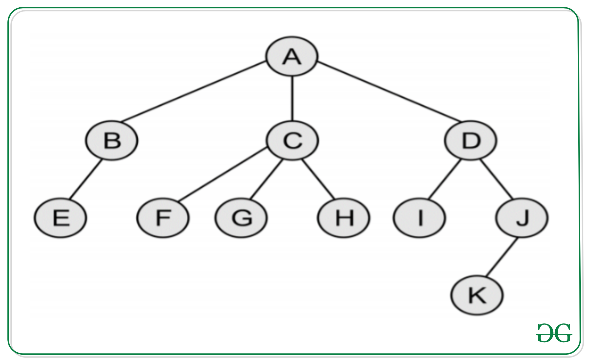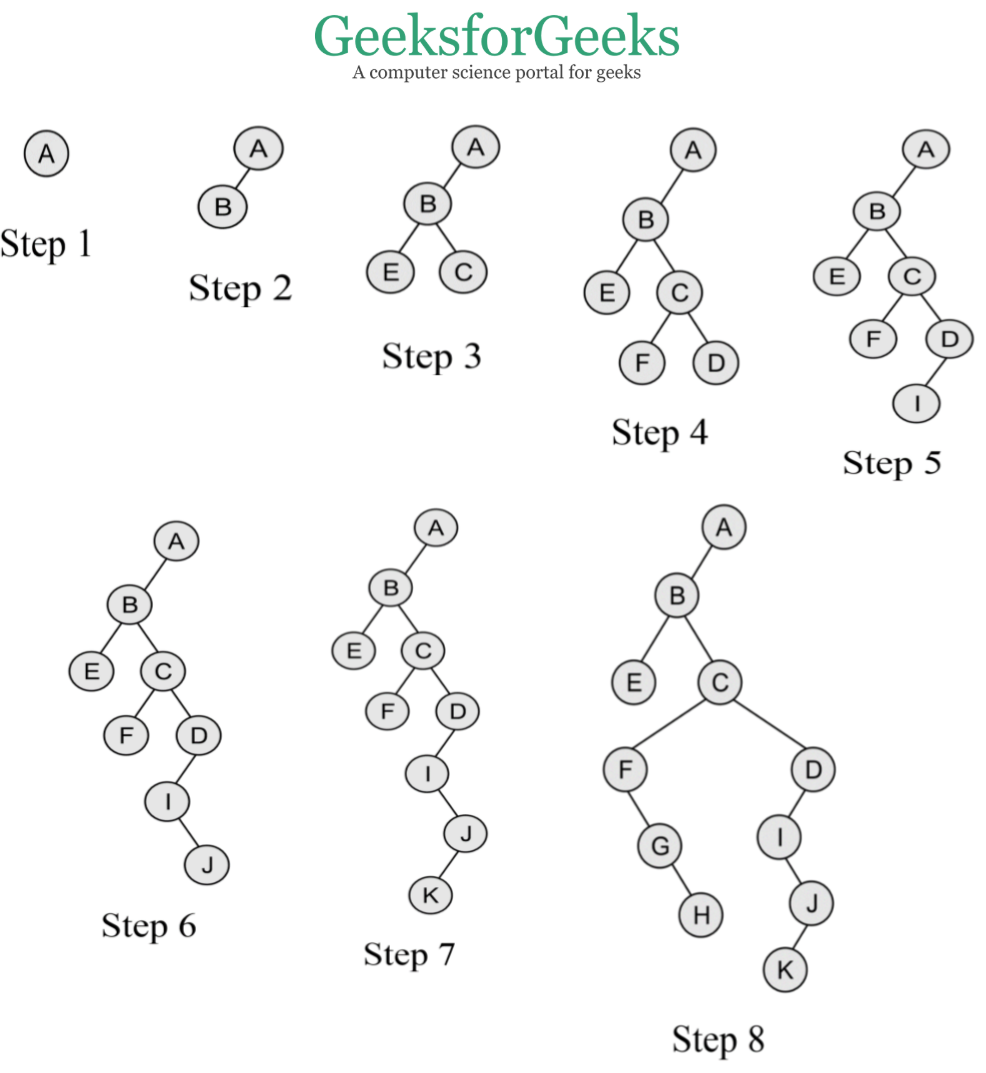# Convert a Generic Tree(N-array Tree) to Binary Tree

• Difficulty Level : Medium
• Last Updated : 29 Oct, 2020

Prerequisite: Generic Trees(N-array Trees)

In this article, we will discuss the conversion of the Generic Tree to a Binary Tree. Following are the rules to convert a Generic(N-array Tree) to Binary Tree:

• The root of the Binary Tree is the Root of the Generic Tree.
• The left child of a node in the Generic Tree is the Left child of that node in the Binary Tree.
• The right sibling of any node in the Generic Tree is the Right child of that node in the Binary Tree.

Examples:
Convert the following Generic Tree to Binary Tree:Below is the Binary Tree of the above Generic Tree:Note: If the parent node has only the right child in the general tree then it becomes the rightmost child node of the last node following the parent node in the binary tree.
In the above example, if node B has the right child node L then in binary tree representation L would be the right child of node D.

Below are the steps for the conversion of Generic Tree to Binary Tree:

1. As per the rules mentioned above, the root node of general tree A is the root node of the binary tree.
2. Now the leftmost child node of the root node in the general tree is B and it is the leftmost child node of the binary tree.
3. Now as B has E as its leftmost child node, so it is its leftmost child node in the binary tree whereas it has C as its rightmost sibling node so it is its right child node in the binary tree.
4. Now C has F as its leftmost child node and D as its rightmost sibling node, so they are its left and right child node in the binary tree respectively.
5. Now D has I as its leftmost child node which is its left child node in the binary tree but doesn’t have any rightmost sibling node, so doesn’t have any right child in the binary tree.
6. Now for I, J is its rightmost sibling node and so it is its right child node in the binary tree.
7. Similarly, for J, K is its leftmost child node and thus it is its left child node in the binary tree.
8. Now for C, F is its leftmost child node, which has G as its rightmost sibling node, which has H as its just right sibling node and thus they form their left, right, and right child node respectively.
My Personal Notes arrow_drop_up